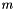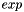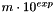UM C API  6.16
lbmsdm_decimal_t_stct Struct Reference

Structure to hold a scaled decimal number. A scaled decimal number consists of a mantissaand an exponent. It represents the value. More...

#include <lbmsdm.h>

int64_t mant

int8_t exp

## Detailed Description

Structure to hold a scaled decimal number. A scaled decimal number consists of a mantissaand an exponent. It represents the value.

The mantissa is represented as a 64-bit signed integer. The exponent is represented as an 8-bit signed integer, and can range from -128 to 127.

## Field Documentation

 int8_t lbmsdm_decimal_t_stct::exp

Exponent.

 int64_t lbmsdm_decimal_t_stct::mant

Mantissa.

The documentation for this struct was generated from the following file: